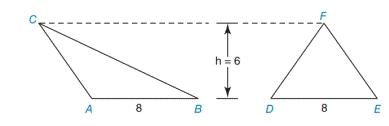Chapter 8.1, Problem 8E### Elementary Geometry for College St...

6th Edition
Daniel C. Alexander + 1 other
ISBN: 9781285195698

#### Solutions

Chapter
Section### Elementary Geometry for College St...

6th Edition
Daniel C. Alexander + 1 other
ISBN: 9781285195698
Textbook Problem
1 views

# Are △ A B C and △ D E F congruent?Exercises 7, 8

To determine

ABC and DEF are congruent or not.

Explanation

1) Approach:

Two polygons are congruent if they are the same size and shape,

(i.e.) If their corresponding angles and sides are equal.

2) Calculation:

Given,

The area of the first triangle (ΔABC):

Here, b=8 and h=6

Area of the triangle

A=12bh

=12(8)(6)

=482

A=24 unit2

The area of the second triangle (ΔDEF):

Here, <

### Still sussing out bartleby?

Check out a sample textbook solution.

See a sample solution

#### The Solution to Your Study Problems

Bartleby provides explanations to thousands of textbook problems written by our experts, many with advanced degrees!

Get Started